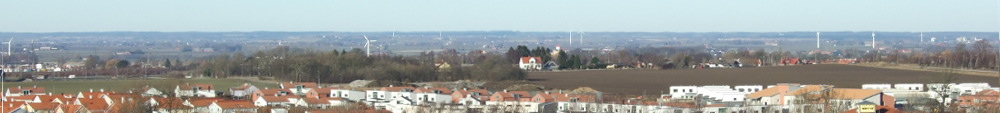## Approximate Dynamic Programming with Applications

### Andreas Wernrud

##### Abstract

This thesis studies approximate optimal control of nonlinear systems. Particular attention is given to global solutions and to the computation of approximately optimal feedback controllers. The solution to an optimal control problem is characterized by the optimal value function. For a large class of problems the optimal value function must satisfy a Hamilton-Jacobi-Bellman type equation. Two common methods for solving such equations are policy iteration and value iteration. Both these methods are studied in this thesis. An approximate policy iteration algorithm is presented for both the continuous and discrete time settings. It is shown that the sequence produced by this algorithm converges monotonically towards the optimal value function. A multivariate polynomial relaxation algorithm is proposed for linearly constrained discrete time optimal control problems with convex cost. Relaxed value iteration is studied for constrained linear systems with convex piecewise linear cost. It is shown how an explicit piecewise linear control law can be computed and how the resulting lookup table can be reduced efficiently. The on-line implementation of receding horizon controllers, even for linear systems, is usually restricted to systems with slow dynamics. One reason for this is that the delay between measurement and actuation introduced by computing the control signal on-line can severely degrade systems with fast dynamics. A method to improve robustness against such delays and other uncertainties is presented. A case study on the control of DC--DC converters is given. Feasibility of a Relaxed Dynamic Programming algorithm is verified by synthesizing controllers for both a step-down converter and a step-up converter. The control performance is evaluated both in simulations and in real experiments.

##### Keywords

Optimal Control, Dynamic Programming, Approximate Optimal Control

PhD Thesis ISRN LUTFD2/TFRT--1082--SE, Department of Automatic Control, Lund University, Sweden, February 2008.